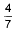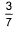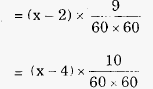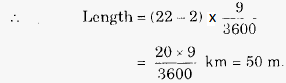# Engineering Maths - Time and Work

>>>>>>>>Time and Work

• A

90• B

81• C

75• D

66• Option : B
• Explanation :of work is completed.
Remaining work = 1-() =Remaining period = 46 - 33 = 13 days
Less work, less men(direct proportion)
Less days, more men(Indirect proportion)
More hours/day, less men(Indirect proportion)
work:Days 13:33 :: 117:x
hrs/day 9:8× 13 × 9 × x = ()33 × 8 × 117
x = (3 × 33 × 8 × 117) / (4 × 13 × 9)
= 198 men
So, additional men to be employed
= 198 - 117 = 81

• A

75 metres• B

60 metres• C

90 metres• D

80 metres• Option : A
• Explanation :
Let L is length of train in km and x is speed of the cyclist, Then
(i) 400L = 42 - x
(ii) 720 L = 12 + x
1120 L = 84
⇒ L = (84 x 1000)/1120 = 75m

• A

2 km/hr• B

1.5 km/hr• C

3 km/hr• D

3.5 km/hr• Option : B
• Explanation :
13/Speed upstream = 5 and
28/Speed downstream = 5
∴ Speed upstream = 13/5 km/hr
and Speed downstream = 28/5 km/hr.• A

75 m, 18 km/hr• B

80 m, 21 km/hr• C

60 m, 20 km/hr• D

50 m, 22 km/hr• Option : D
• Explanation :
in each case, train has to travel its own length, to pass each man.
If x km/hr is speed of the train, then
Length = Relative speed x Time∴ 9x - 18 = 10x - 40
⇒ x = 22km/hr.• A

100 m• B

125 m• C

150 m• D

250 m• Option : C
• Explanation :Related Quiz.
Time and Work